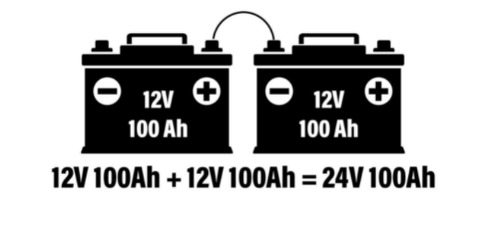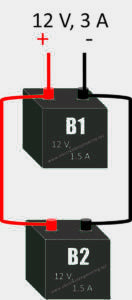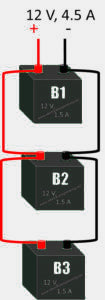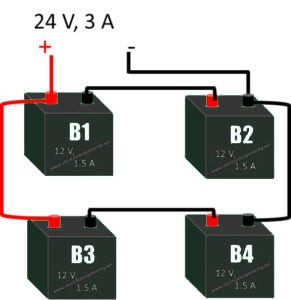https://www.electricalengineering.xyz/battery/3-types-of-battery-connections/

• 1 Basics of Batteries
• 2 Series connection
• 2.1 Steps to connect two batteries in series
• 2.2 Steps to connect 3 Batteries in Series
• 3 Parallel Connection
• 3.1 Steps to connect two batteries in Parallel
• 3.2 Steps to connect three batteries in parallel
• 4 Series-Parallel Battery Connections
• 5 Steps to connect four batteries in Series-Parallel

## Basics of Batteries

Things you should know:

## 2.Series connection

Connecting two or more batteries in series can increase the voltage of the battery system, but the ampere hour rating remains the same. For example, two 12V 100AH ​​batteries are connected in series to a 24V battery system, the bank still has a total capacity rating of 100 AhRemember, when connecting in series, each battery needs to have the same voltage and rated capacity, otherwise the battery will eventually be damaged

Procedure: Connect first [battery- ] with the second [battery +]. Continue this connection for all batteries. Take the output from [battery+] of first and [battery- ] of last one.

### Steps to connect two batteries in series

Let’s name them B1 and B2.

1. Connect the [ B1 - ] to the [B2+]
2. Connect a wire W1 (red wire) to [B1+]  .
3. Connect W3 (black wire) to[ B2 - ]
4. Take the output from W1 and W3

The figure below illustrates this.

###### Steps to connect 3 Batteries in Series

Let’s name B1, B2, B3.

1. Connect [ B1 - ] to [ B2 + ]
2. Connect [ B2 - ] to [ B3 + ].
3. Take the output from [ B1 +].
4. Take the output from [ B3 - ].Usage Example:

Let’s assume B1 = 12 V, B2 = 12 V and B3 = 12V

Now using the series connection, you can obtain 36 V.

## Parallel Connection

Parallel connection involves connecting 2 or more batteries together, which increases the ampere-hour capacity of the battery bank, but the voltage remains the same. .

Procedure:  Connect all anode terminals to a common terminal, and all cathodes to each other on the other terminal.

### Steps to connect two batteries in Parallel

Let’s name them B1 and B2.

1. Use connecting wire for joining anode to a common terminal.
2. Use other connecting wire for joining cathode to the other terminal.
3. Take one output from the anode.
4. Take the second output from the cathode.

The above connection ads up the current output. Let’ assume B1 = 1.5 A and B2 = 1.5 A

Then output Amps = 3 A while voltage remains constant.\### Steps to connect three batteries in parallel

Let’s name the batteries B1, B2, and B3.

1.Use connecting wire for joining anode to a common terminal.

2.Use other connecting wire for joining cathode to the other terminal.

3.Take one output from the anode.

4.Take the second output from the cathode.

The above connection lumps the amp-hours  taken from batteries. Let’s assume B1 = 100Ah, B2 = 100Ah and B3 = 100Ah. The final battery capacity = 300Ah.## Steps to connect four batteries in Series-Parallel

Let’s name them B1, B2, B3, and B4 and each rated at 12 V@100 Ah. We are willing to get 24 V@200 Ah from both. First,Series B1, B2 ;Second,series B3, B4;then connect series B1 and B2  in parallel with series B3 and B4 ..

1. Connect cathode of B1 to anode of B2.
2. Connect cathode of B3 to anode of B4.
3. Connect cathode of B1 to anode of B3.
4. Connect cathode of B1 to cathode of B3.
5. Take output from [ B1 + ] cathode and [ B2 - ].